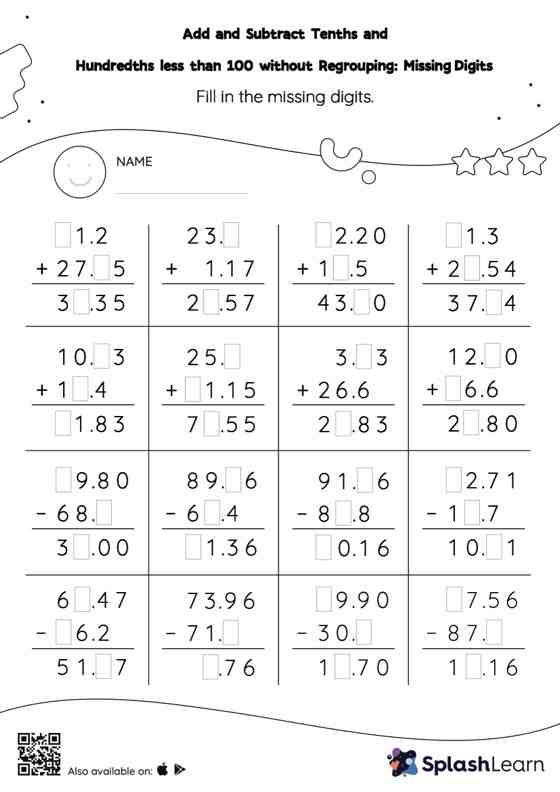# Add and Subtract Tenths and Hundredths less than 100 without Regrouping: Missing Digits Worksheet

Home > Add and Subtract Tenths and Hundredths less than 100 without Regrouping: Missing DigitsGive your little one some practice building addition and subtraction skills with this add and subtract tenths and hundredths less than 100 without regrouping worksheet. Students use the relationship between addition and subtraction to find the missing number while adding and subtracting decimals. They do not need to regroup the numbers in add and subtract tenths and hundredths less than 100 without regrouping worksheet to get to the result. In this worksheet, numbers are laid one on top of another (vertical format). This encourages students to use the inherent place value structure to solve the problems.